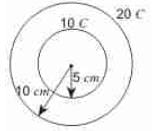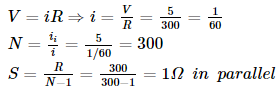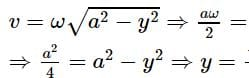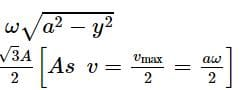JEE  >  BITSAT Physics Test - 4

# BITSAT Physics Test - 4

Test Description

## 40 Questions MCQ Test BITSAT Mock Tests Series & Past Year Papers | BITSAT Physics Test - 4

BITSAT Physics Test - 4 for JEE 2023 is part of BITSAT Mock Tests Series & Past Year Papers preparation. The BITSAT Physics Test - 4 questions and answers have been prepared according to the JEE exam syllabus.The BITSAT Physics Test - 4 MCQs are made for JEE 2023 Exam. Find important definitions, questions, notes, meanings, examples, exercises, MCQs and online tests for BITSAT Physics Test - 4 below.
Solutions of BITSAT Physics Test - 4 questions in English are available as part of our BITSAT Mock Tests Series & Past Year Papers for JEE & BITSAT Physics Test - 4 solutions in Hindi for BITSAT Mock Tests Series & Past Year Papers course. Download more important topics, notes, lectures and mock test series for JEE Exam by signing up for free. Attempt BITSAT Physics Test - 4 | 40 questions in 50 minutes | Mock test for JEE preparation | Free important questions MCQ to study BITSAT Mock Tests Series & Past Year Papers for JEE Exam | Download free PDF with solutions
 1 Crore+ students have signed up on EduRev. Have you?
BITSAT Physics Test - 4 - Question 1

### A solenoid has an inductance of 60 henrys and a resistance of 30 ohms. If it is connected to a 100 volt battery, how long will it take for the current to reach e-1/e=63.2% of its final value

BITSAT Physics Test - 4 - Question 2

### The force acting between proton and proton inside the nucleus is

BITSAT Physics Test - 4 - Question 3

### The main source of solar energy is

BITSAT Physics Test - 4 - Question 4

The north pole of a long horizontal bar magnet is being brought closer to a vertical conducting plane, along the perpendicular direction. The induced current in the conducting plane is

Detailed Solution for BITSAT Physics Test - 4 - Question 4

As per Lenz's law, induced current must be anticlockwise, so as to develop north polarity to oppose the incoming north pole.

BITSAT Physics Test - 4 - Question 5

The electrical resistance of a mercury column in a cylindrical container is R. When the same mercury is poured into another cylindrical container twice the radius of cross-section, the resistance of mercury column now is

BITSAT Physics Test - 4 - Question 6

Consider the two following statements A and B and identify the correct choice given in the answers; (A) In photovoltaic cells the photoelectric current produced is not proportional to the, intensity of incident light. (B) in gas filled photoemissive cells, the velocity of photoelectrons depends on the wavelength of the incident radiation.

BITSAT Physics Test - 4 - Question 7

A body of 2 gm carrying a charge of 0·1 μ C starts from rest at the positive plate and moves to the negative plate of a parallel plate condenser connected to a voltage supply of 30KV. The final velocity is

BITSAT Physics Test - 4 - Question 8

A block rests on a rough inclined plane making an angle of 30 with the horizontal. The coefficient of static friction between block and the plane is 0.8. If the frictional force on the block is 10 N, the mass of block is (g=10m/s2)

BITSAT Physics Test - 4 - Question 9

In potentiometer experiment a cell of e.m.f. 1.5V connected in the secondary circuit gives a balancing length of 165cm of the wire. If a resistance of 5 Ω is connected parallel to the cell the balancing length of the wire is 150cm. The internal resistance of the cell is

BITSAT Physics Test - 4 - Question 10

In a closed room, heat transfer takes place by

BITSAT Physics Test - 4 - Question 11

Two concentric uniformly charged spheres of radius 5cm and 10cm are arranged as shown in the figure. Potential difference between the spheres is:BITSAT Physics Test - 4 - Question 12

If one mole of monoatomic gas (γ = 5/3) is mixed with one mole of diatomic gas (γ = 7/5), the value of γ for the mixture is

BITSAT Physics Test - 4 - Question 13

Choose the correct statement from the following : Weightlessness of an astronaut moving in a satellite is a situation of

BITSAT Physics Test - 4 - Question 14

Two tangent galvanometers having coils of the same radius are connected in series. A current flowing in them produces deflections of 60o and 45orespectively. The ratio of the number of turns in the coils is

BITSAT Physics Test - 4 - Question 15

The range of voltmeter of resistance 300Ω is 5V. The resistance to be connected to convert it is an ammeter of range 5A is

Detailed Solution for BITSAT Physics Test - 4 - Question 15BITSAT Physics Test - 4 - Question 16

A capillary tube when immersed vertically in a liquid records a rise of 3 mm. If the tube is immersed in the liquid at an angle of 60o with the vertical, then height of the liquid in the capillary tube will be

BITSAT Physics Test - 4 - Question 17

In a liquid flowing through a pipe, the pressure difference between its ends is made half and radius is doubled. If the initial rate of flow of liquid was Q, then new rate of flow of liquid through the pipe is

BITSAT Physics Test - 4 - Question 18

Shearing stress produces a change in

BITSAT Physics Test - 4 - Question 19

Particle nature and wave nature of electromagnetic waves and electrons be shown by

BITSAT Physics Test - 4 - Question 20

A body falling from a high Minaret travels 40 metres in the last 2 seconds of its fall to ground. Height of Minaret in metres is (take g=10m/s2)

BITSAT Physics Test - 4 - Question 21

A mass of 100 gm is tied to one end of string 2 m long. The body is revolving in a horizontal circle making a maximum of 200 revolutions per min. The other end of the string is fixed at the centre of the circle of revolution. The maximum tension that the string can bear is (approximately)

BITSAT Physics Test - 4 - Question 22

A circular road of radius 1000 m has banking angle 45o. The maximum safe speed of a car having mass 2000 kg will be, if the coefficient of friction between tyre and road is 0.5

BITSAT Physics Test - 4 - Question 23

The velocity of a body of rest mass m₀ is √3/2c (where c is the velocity of light in vacuum). Then mass of this body is

BITSAT Physics Test - 4 - Question 24

In an astronomical telescope, the focal lengths of objective lens and eye-piece are 180 cm and 6 cm respectively. In normal adjustment, magnifying power of the astronomical telescope will be

BITSAT Physics Test - 4 - Question 25

A body executes SHM. The P.E., the K.E. and total energy (T.E.) are measured as a function of displacement x. Which of the following statement is true.

BITSAT Physics Test - 4 - Question 26

In an orbital motion, the angular momentum vector is

BITSAT Physics Test - 4 - Question 27

The rotational kinetic energy of a body is E and its moment of inertia is I. The angular momentum is

BITSAT Physics Test - 4 - Question 28

A spherical shell has mass M and radius R. Moment of inertia about its diameter will be

BITSAT Physics Test - 4 - Question 29

An electric kettle has two heating coils. When one of the coils is connected to an ac source the water in the kettle boils in 10 minutes. When the other coil is used the water boils in 40 minutes. If both the coils are connected in parallel, the time taken by the same quantity of water to boil will be

BITSAT Physics Test - 4 - Question 30

In isothermic process, which statement is wrong

BITSAT Physics Test - 4 - Question 31

A monoatomic gas of n moles is heated from temperature T₁ to T₂ under two different conditions (i) constant volume (ii) constant pressure. The change in internal energy of the gas

BITSAT Physics Test - 4 - Question 32

Which physical quantities have same dimensions?

BITSAT Physics Test - 4 - Question 33

Light travels in two media A and B with speed 2*10 8 ms− 1 and 2.265* 108 ms−1 respectively. The sine of critical angle C, between them is

BITSAT Physics Test - 4 - Question 34

In a biprism expt., by using light of wavelength 5000 Å, 5 mm wide fringes are obtained on a screen 1.0 m away from coherent sources. The separation between two coherent sources is

BITSAT Physics Test - 4 - Question 35

The ray of light

BITSAT Physics Test - 4 - Question 36

A particle has displacement y given by y = 3 sin (5 π t + φ), where y is in metre and t is in second. What are frequency and period of motion?

BITSAT Physics Test - 4 - Question 37

A particle starts S.H.M. from the mean position. Its amplitude is A and time period is T. At the time when its speed is half of the maximum speed, its displacement y is

Detailed Solution for BITSAT Physics Test - 4 - Question 37BITSAT Physics Test - 4 - Question 38

The displacement of a wave is given by the equation: y = 10 sin(2π t/30 + α). If the displacement is 5 cm at t = 0, then angle described by the wave at t = 7.5 s will be

BITSAT Physics Test - 4 - Question 39

The quantity that is not conserved in an inelastic collision is

BITSAT Physics Test - 4 - Question 40

in an explosion a body breaks up into two pieces of unequal masses. In this

## BITSAT Mock Tests Series & Past Year Papers

2 videos|15 docs|70 tests
 Use Code STAYHOME200 and get INR 200 additional OFF Use Coupon Code
Information about BITSAT Physics Test - 4 Page
In this test you can find the Exam questions for BITSAT Physics Test - 4 solved & explained in the simplest way possible. Besides giving Questions and answers for BITSAT Physics Test - 4, EduRev gives you an ample number of Online tests for practice

## BITSAT Mock Tests Series & Past Year Papers

2 videos|15 docs|70 tests